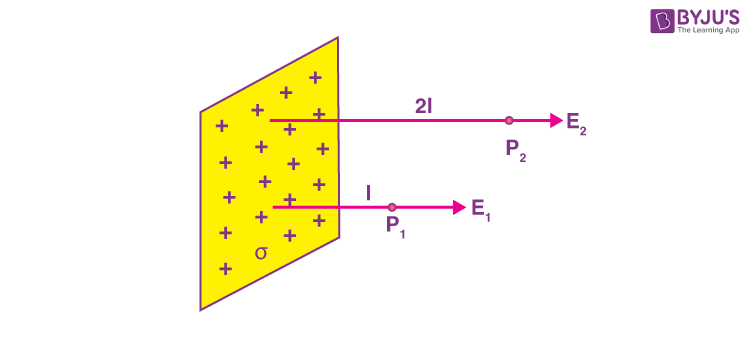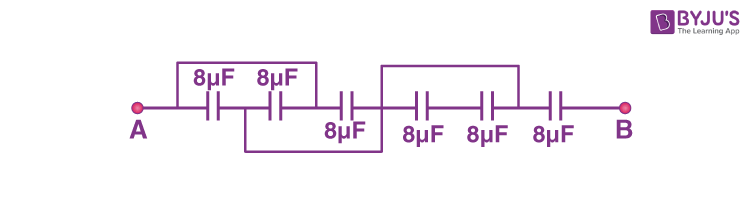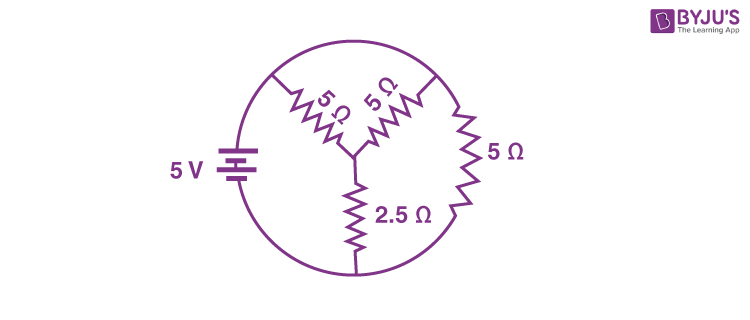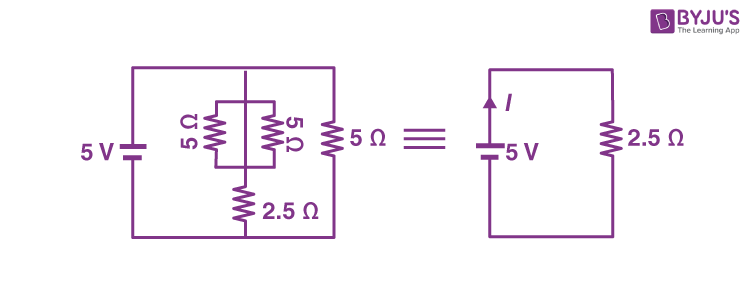Win up to 100% scholarship on Aakash BYJU'S JEE/NEET courses with ABNAT Win up to 100% scholarship on Aakash BYJU'S JEE/NEET courses with ABNAT

# JEE Main 2022 June 25 – Shift 1 Physics Question Paper with Solutions

## JEE Main 2022 June 25 – Shift 1 Physics Question Paper with Solutions

JEE Main 2022 June 25 – Shift 1 Physics Question Paper with Solutions

SECTION – A

Multiple Choice Questions: This section contains 20 multiple choice questions. Each question has 4 choices (1), (2), (3) and (4), out of which ONLY ONE is correct.

1. If

$$\begin{array}{l}Z=\frac{A^2B^3}{C^4}\end{array}$$
, then the relative error in Z will be

(A)

$$\begin{array}{l}\frac{\Delta A}{A}+\frac{\Delta B}{B}+\frac{\Delta C}{C}\end{array}$$

(B)

$$\begin{array}{l}\frac{2\Delta A}{A}+\frac{3\Delta B}{B}-\frac{4\Delta C}{C}\end{array}$$

(C)

$$\begin{array}{l}\frac{2\Delta A}{A}+\frac{3\Delta B}{B}+\frac{4\Delta C}{C}\end{array}$$

(D)

$$\begin{array}{l}\frac{\Delta A}{A}+\frac{\Delta B}{B}-\frac{\Delta C}{C}\end{array}$$

Sol.

$$\begin{array}{l}Z=\frac{A^{2}B^{3}}{C^{4}}\end{array}$$

$$\begin{array}{l}\frac{\Delta Z}{Z}=\frac{2\Delta A}{A}+3\times\frac{\Delta B}{B}+ \frac{4\Delta C}{C}\end{array}$$

2.

$$\begin{array}{l}\vec{A}\end{array}$$
is a vector quantity such that
$$\begin{array}{l}|\vec{A}| \end{array}$$
= non-zero constant. Which of the following expression is true for
$$\begin{array}{l}\vec{A}\end{array}$$
?

(A)

$$\begin{array}{l}\vec{A}\cdot\vec{A} = 0\end{array}$$

(B)

$$\begin{array}{l}\vec{A}\times\vec{A} < 0\end{array}$$

(C)

$$\begin{array}{l}\vec{A}\times\vec{A} = 0\end{array}$$

(D)

$$\begin{array}{l}\vec{A}\times\vec{A} > 0\end{array}$$

Sol.

$$\begin{array}{l}\vec{A}\times\vec{A} = A\times A\times \sin 0^{\circ}\end{array}$$

= 0

3. Which of the following relations is true for two unit vector

$$\begin{array}{l}\hat{A}~\text{and}~\hat{B}\end{array}$$
making an angle θ to each other?

(A)

$$\begin{array}{l}|\hat{A}+\hat{B}|=|\hat{A}-\hat{B}|tan\frac{\theta}{2}\end{array}$$

(B)

$$\begin{array}{l}|\hat{A}-\hat{B}|=|\hat{A}+\hat{B}|tan\frac{\theta}{2}\end{array}$$

(C)

$$\begin{array}{l}\left| \hat{A}+\hat{B}\right|= \left|\hat{A}-\hat{B}\right|\cos\frac{\theta}{2}\end{array}$$

(D)

$$\begin{array}{l}\left| |\hat{A}-\hat{B}\right|=\left| \hat{A}+\hat{B}\right|\cos\frac{\theta}{2}\end{array}$$

Sol.

$$\begin{array}{l}\left| \hat{A}-\hat{B}\right|=2\sin\left ( \frac{\theta}{2} \right )\end{array}$$

and,

$$\begin{array}{l}\left| \hat{A}+\hat{B}\right|=2\cos\left ( \frac{\theta}{2} \right )\end{array}$$

$$\begin{array}{l}\frac{\left| \hat{A}-\hat{B}\right|}{{\left| \hat{A}+\hat{B}\right|}}=\tan\left ( \frac{\theta}{2} \right )\end{array}$$

4. If force

$$\begin{array}{l}\vec{F}=3\hat{i}+4\hat{j}-2\hat{k} \end{array}$$
acts on a particle having position vector
$$\begin{array}{l}2\hat{i}+\hat{j}+2\hat{k} \end{array}$$
then, the torque about the origin will be

(A)

$$\begin{array}{l}3\hat{i}+4\hat{j}-2\hat{k} \end{array}$$

(B)

$$\begin{array}{l}-10\hat{i}+10\hat{j}+5\hat{k} \end{array}$$

(C)

$$\begin{array}{l}10\hat{i}+5\hat{j}-10\hat{k} \end{array}$$

(D)

$$\begin{array}{l}10\hat{i}+\hat{j}-5\hat{k} \end{array}$$

Sol.

$$\begin{array}{l}\vec{\tau}=\vec{r}\times\vec{F}\end{array}$$
$$\begin{array}{l}=\left ( 2\hat{i}+\hat{j}+2\hat{k} \right )\times\left ( 3\hat{i}+4\hat{j}-2\hat{k} \right )\end{array}$$
$$\begin{array}{l}=-10\hat{i}+10\hat{j}+5\hat{k}\end{array}$$

5. The height of any point P above the surface of earth is equal to diameter of earth. The value of acceleration due to gravity at point P will be (Given g = acceleration due to gravity at the surface of earth).

(A)

$$\begin{array}{l}\frac{g}{2}\end{array}$$

(B)

$$\begin{array}{l}\frac{g}{4}\end{array}$$

(C)

$$\begin{array}{l}\frac{g}{3}\end{array}$$

(D)

$$\begin{array}{l}\frac{g}{9}\end{array}$$

Sol.h = 2R

$$\begin{array}{l}{g}\ ‘ =\frac{GM}{\left ( R+h \right )^2}\end{array}$$
$$\begin{array}{l}\frac{GM}{9R^2}\end{array}$$
$$\begin{array}{l}=\frac{g}{9}\end{array}$$

6. The terminal velocity (vt) of the spherical rain drop depends on the radius (r) of the spherical rain drop as

(A) r1/2 (B) r

(C) r2 (D) r3

Sol.

$$\begin{array}{l}6\pi\eta v_tr=\frac{4}{3}\pi r^3\left ( \rho-\sigma \right )g\end{array}$$

vt = Cr2 where C is a constant

or vtr2

7. The relation between root mean square speed (vrms) and most probable speed (vp) for the molar mass M of oxygen gas molecule at the temperature of 300 K will be

(A)

$$\begin{array}{l}v_{rms}=\sqrt{\frac{2}{3}}v_p\end{array}$$

(B)

$$\begin{array}{l}v_{rms}=\sqrt{\frac{3}{2}}v_p\end{array}$$

(C) vrms = vp

(D)

$$\begin{array}{l}v_{rms}=\sqrt{\frac{1}{3}}v_p\end{array}$$

Sol.

$$\begin{array}{l}v_{rms}=\sqrt{\frac{3RT}{M}}\end{array}$$
$$\begin{array}{l}v_{p}=\sqrt{\frac{2RT}{M}}\end{array}$$

$$\begin{array}{l}v_{rms}=\sqrt{\frac{3}{2}}v_p\end{array}$$

⇒ option (B)

8. In the figure, a very large plane sheet of positive charge is shown. P1 and P2 are two points at distance l and 2l from the charge distribution. If σ is the surface charge density, then the magnitude of electric fields E1 and E2 and P1 and P2 respectively are(A)

$$\begin{array}{l}E_1=\frac{\sigma}{\varepsilon_0},E_2=\frac{\sigma}{2\varepsilon_0}\end{array}$$

(B)

$$\begin{array}{l}E_1=\frac{2\sigma}{\varepsilon_0},E_2=\frac{\sigma}{\varepsilon_0}\end{array}$$

(C)

$$\begin{array}{l}E_1=E_2=\frac{\sigma}{2\varepsilon_0}\end{array}$$

(D)

$$\begin{array}{l}E_1=E_2=\frac{\sigma}{\varepsilon_0}\end{array}$$

Sol. For an infinite charged plane

$$\begin{array}{l}E=\frac{\sigma}{2\varepsilon_0}\end{array}$$
for any value of l

$$\begin{array}{l}E_1=E_2=\frac{\sigma}{2\varepsilon_0}\end{array}$$

⇒ option (C)

9. Match List-I with List-II

List-I

List-II

(A)

AC generator

(I)

Detects the presence of current in the circuit

(B)

Galvanometer

(II)

Converts mechanical energy into electrical energy

(C)

Transformer

(III)

Works on the principle of resonance in AC circuit

(D)

Metal detector

(IV)

Changes an alternating voltage for smaller or greater value

Choose the correct answer from the options given below

(A) (A) – (II), (B) – (I), (C) – (IV), (D) – (III)

(B) (A) – (II), (B) – (I), (C) – (III), (D) – (IV)

(C) (A) – (III), (B) – (IV), (C) – (II), (D) – (I)

(D) (A) – (III), (B) – (I), (C) – (II), (D) – (IV)

Sol.

AC generator

Converts mechanical energy into electrical energy

Galvanometer

Detects the presence of current in the circuit

Transformer

Change AC voltage for smaller or greater value

Metal detector

Works on the principle of resonance in AC circuit

⇒ Option (A) is correct

10. A long straight wire with a circular cross-section having radius R, is carrying a steady current I. The current I is uniformly distributed across this cross-section. Then the variation of magnetic field due to current I with distance r (r<R) from its centre will be

(A) Br2

(B) Br

(C)

$$\begin{array}{l}B\propto\frac{1}{r^2}\end{array}$$

(D)

$$\begin{array}{l}B\propto\frac{1}{r}\end{array}$$

Sol.

$$\begin{array}{l}\int\overline{B}\cdot\overline{dl}=\mu_0l_{in} \end{array}$$

$$\begin{array}{l}B\times2\pi r=\frac{\mu_0l}{\pi R^2}\times\pi r^2\end{array}$$

Br

⇒ option (B) is correct

11. If wattless current flows in the AC circuit, then the circuit is :

(A) Purely Resistive circuit

(B) Purely Inductive circuit

(C) LCR series circuit

(D) RC series circuit only

Sol. For wattless current to flow in AC circuit the circuit will bePurely Inductive circuit

12. The electric field in an electromagnetic wave is given by E = 56.5 sinω(tx/c) NC–1. Find the intensity of the wave if it is propagating along x-axis in the free space.

(Given ∈0 = 8.85 × 10–12C2N–1m–2)

(A) 5.65 Wm–2

(B) 4.24 Wm–2

(C) 1.9 × 10–7 Wm–2

(D) 56.5 Wm–2

Sol.

$$\begin{array}{l}I=\frac{1}{2}\varepsilon_0 E_0^2c\end{array}$$
$$\begin{array}{l}=\frac{1}{2}8.5\times10^{-12}\times\left ( 56.5 \right )^2\times3\times10^8\end{array}$$

= 4.24 W/m2

13. The two light beams having intensities I and 9I interfere to produce a fringe pattern on a screen. The phase difference between the beams is π/2 at point P and π at point Q. Then the difference between the resultant intensities at P and Q will be:

(A) 2I

(B) 6I

(C) 5I

(D) 7I

Sol.

$$\begin{array}{l}I_P=I+9I+2\sqrt{I\times9I}cos\frac{\pi}{2}=10I\end{array}$$
$$\begin{array}{l}I_Q=I+9I+2\sqrt{I\times9I}cos\pi=4I\end{array}$$

So, IPIQ = 6I

14. A light wave travelling linearly in a medium of dielectric constant 4, incidents on the horizontal interface separating medium with air. The angle of incidence for which the total intensity of incident wave will be reflected back into the same medium will be :

(Given : relative permeability of medium μr= 1)

(A) 10° (B) 20°

(C) 30° (D) 60°

Sol.

$$\begin{array}{l}n=\sqrt{k\mu}=2\end{array}$$
(n⇒ refractive index)

So for TIR

$$\begin{array}{l}\theta>\sin^{-1}\left (\frac{1}{n}\right )\end{array}$$

θ> 30

Only option is 60°

15. Given below are two statements :

Statement I: Davisson-Germer experiment establishes the wave nature of electrons.

Statement II: If electrons have wave nature, they can interfere and show diffraction.

In the light of the above statements choose the correct answer from the option given below :

(A) Both statement I and statement II are true.

(B) Both statement I and statement II are false.

(C) Statement I is true but statement II is false.

(D) Statement I is false but statement II is true.

Sol. Davisson-Germer experiment is done and establishes the wave nature of electrons. Interference and diffraction establishes wave nature.

16. The ratio for the speed of the electron in the 3rd orbit of He+ to the speed of the electron in the 3rd orbit of hydrogen atom will be :

(A) 1 : 1

(B) 1 : 2

(C) 4 : 1

(D) 2 : 1

Sol. We know that

$$\begin{array}{l}V\propto\frac{Z}{n}\end{array}$$

⇒ Required ratio

$$\begin{array}{l}=\frac{\frac{2}{3}}{\frac{1}{3}}\end{array}$$

= 2 : 1

17. The photodiode is used to detect the optical signals. These diodes are preferably operated in reverse biased mode because :

(A) fractional change in majority carriers produce higher forward bias current

(B) fractional change in majority carriers produce higher reverse bias current

(C) fractional change in minority carriers produce higher forward bias current

(D) fractional change in minority carriers produce higher reverse bias current

Sol. A photodiode is reverse biased. When light falling on it produces charge carriers, the fractional change, in minority carriers is high since the original current is very small.

18. A signal of 100 THz frequency can be transmitted with maximum efficiency by :

(A) Coaxial cable

(B) Optical fibre

(C) Twisted pair of copper wires

(D) Water

Sol. Optical fibres supports frequency of electromagnetic waves in the range 1014 Hz to 1015 Hz.

19. The difference of speed of light in the two media A and BA – υB) is 2.6 × 107 m/s. If the refractive index of medium B is 1.47, then the ratio of refractive index of medium B to medium A is: (Given: speed of light in vacuum C = 3 × 108ms–1)

(A) 1.303

(B) 1.318

(C) 1.13

(D) 0.12

Sol. Speed of light in a medium

$$\begin{array}{l}=\frac{c}{n}\end{array}$$

⇒ According to given information,

$$\begin{array}{l}\frac{c}{n_A}-\frac{c}{n_B}=2.6\times10^7\end{array}$$

$$\begin{array}{l}\frac{n_B}{n_A}-1=\frac{2.6\times10^7}{3\times10^8}\times n_B\end{array}$$

$$\begin{array}{l}\frac{n_B}{n_A}\simeq 1.13\end{array}$$

20. A teacher in his physics laboratory allotted an experiment to determine the resistance (G) of a galvanometer. Students took the observations for

$$\begin{array}{l}\frac{1}{3}\end{array}$$
deflection in the galvanometer. Which of the below is true for measuring value of G?

(A)

$$\begin{array}{l}\frac{1}{3}\end{array}$$
deflection method cannot be used for determining the resistance of the galvanometer.

(B)

$$\begin{array}{l}\frac{1}{3}\end{array}$$
deflection method can be used and in this case the G equals to twice the value of shunt resistance(s)

(C)

$$\begin{array}{l}\frac{1}{3}\end{array}$$
deflection method can be used and in this case, the G equals to three times the value of shunt resistance(s)

(D)

$$\begin{array}{l}\frac{1}{3}\end{array}$$
deflection method can be used and in this case the G value equals to the shunt resistance(s)

Sol. The circuit for the given situation is:Since G and S are in parallel,

$$\begin{array}{l}\frac{i}{3}\times G=\frac{2i}{3}\times S\end{array}$$

G = 2S

G equals twice the value of shunt resistance.

SECTION – B

Numerical Value Type Questions: This section contains 10 questions. In Section B, attempt any five questions out of 10. The answer to each question is a NUMERICAL VALUE. For each question, enter the correct numerical value (in decimal notation, truncated/rounded-off to the second decimal place; e.g. 06.25, 07.00, –00.33, –00.30, 30.27, –27.30) using the mouse and the on-screen virtual numeric keypad in the place designated to enter the answer.

1. A uniform chain of 6 m length is placed on a table such that a part of its length is hanging over the edge of the table. The system is at rest. The co-efficient of static friction between the chain and the surface of the table is 0.5, the maximum length of the chain hanging from the table is ____m.

Sol. (x)gλ = μ(6 – x) gλ where x is length of hanging part

x = 3 – 0.5x

x = 2 m

2. A 0.5 kg block moving at a speed of 12 ms–1compresses a spring through a distance 30 cm when its speed is halved. The spring constant of the spring will be ___ Nm–1.

Sol.

$$\begin{array}{l}\frac{1}{2}mV^2=\frac{1}{2}kx^2+\frac{1}{2}m\left (\frac{v}{2} \right )^2\end{array}$$
$$\begin{array}{l}\Rightarrow \frac{3}{8}mv^2=\frac{1}{2}kx^2\end{array}$$
$$\begin{array}{l}\Rightarrow k=\frac{3}{4}\times\frac{1}{2}\times\frac{144}{9}\times100 \end{array}$$

= 600

⇒ 600

3. The velocity of upper layer of water in a river is 36 kmh–1. Shearing stress between horizontal layers of water is 10–3 Nm–2. Depth of the river is ____ m. (Co-efficient of viscosity of water is 10–2Pa.s)

Sol.

$$\begin{array}{l}F=-\eta A\frac{du}{dx}\end{array}$$

$$\begin{array}{l}10^{-3}=10^{-2}\times\frac{10}{h}\end{array}$$

$$\begin{array}{l}h=\frac{10^{-1}}{10^{-3}}m=100~m\end{array}$$

⇒ (100)

4. A steam engine intakes 50 g of steam at 100°C per minute and cools it down to 20°C. If latent heat of vaporization of steam is 540 cal g–1, then the heat rejected by the steam engine per minute is
___ × 103 cal.

(Given : specific heat capacity of water : 1 cal
g–1 °C–1)

Sol. ΔQrej = 50 × 540 + 50 × 1 × (100 – 20)

= 50 × [540 + 80]

= 50 × 620

= 31000 cal

= 31 × 103cal

5. The first overtone frequency of an open organ pipe is equal to the fundamental frequency of a closed organ pipe. If the length of the closed organ pipe is 20 cm. The length of the open organ pipe is ___ cm.

Sol.

$$\begin{array}{l}2\times\left ( \frac{V}{2L_0} \right )|=\left ( \frac{V}{4L_C} \right )\end{array}$$

⇒ L0 = 4LC

= 4 × 20

= 80 cm

6. The equivalent capacitance between points A and B in below shown figure will be _______μF.Sol.

$$\begin{array}{l}C_{eq}=\frac{\left ( 3\times8 \right )\times8}{\left ( 3\times8 \right )+8}\end{array}$$
$$\begin{array}{l}=\frac{24\times8}{32}\end{array}$$

= 6 μF

7. A resistor develops 300 J of thermal energy in 15 s, when a current of 2 A is passed through it. If the current increases to 3 A, the energy developed in 10 s is _______ J.

Sol.

R = 5 Ω

Now

$$\begin{array}{l}I_2^2Rt_2\end{array}$$

= 9 × 5 × 10

= 450 J

8. The total current supplied to the circuit as shown in figure by the 5 V battery is _________A.Sol. The equivalent circuit is$$\begin{array}{l}I=\frac{5}{2.5}=2~A\end{array}$$

9. The current in a coil of selfinductance 2.0H is increasing according to I = 2sin(t2)A. The amount of energy spent during the period when current changes from 0 to 2A is _______ J.

Sol.

$$\begin{array}{l}U=\frac{1}{2}LI^2\end{array}$$
$$\begin{array}{l}=\frac{1}{2}2\times2^2=4 J \end{array}$$

10. A force on an object of mass 100 g is

$$\begin{array}{l}\left ( 10\hat{i}+5\hat{j} \right )N\end{array}$$
. The position of that object at t=2s is
$$\begin{array}{l}\left ( a\hat{i}+b\hat{j} \right )m\end{array}$$
after starting from rest. The value of
$$\begin{array}{l}\frac{a}{b}\end{array}$$
will be ______.

Sol.

$$\begin{array}{l}\vec{F} = m\vec{a}\end{array}$$

$$\begin{array}{l}\vec{a}=100\hat{i}+50\hat{j}\end{array}$$

So

$$\begin{array}{l}\vec{S}=\frac{1}{2\vec{a}}t^2\end{array}$$
$$\begin{array}{l}\frac{1}{2}\left ( 100\hat{i}+50\hat{j} \right )2^2\end{array}$$

=

$$\begin{array}{l}200\hat{i}+100\hat{j}\end{array}$$
m

soa = 200 m and b = 100 m

so

$$\begin{array}{l}\frac{a}{b}=2\end{array}$$